# Consistency Checking of the IEC 61508 PFH Formulas and New Formulas Proposal Based on the Markovian Approach

Consistency Checking of the IEC 61508 PFH Formulas and New Formulas Proposal Based on the Markovian Approach
Institute of Applied Sciences and Techniques, University of 20 Août 1955, Skikda 21000, Algeria

Department of Process Engineering, University of 20 Août 1955, Skikda 21000, Algeria

Department of Mechanical and Industrial Engineering, Norwegian University of Science and Technology, Verkstedteknisk, 517, Gløshaugen, Richard Birkeland 2B, Trondheim, Norway

Corresponding Author Email:
h.omeiri@univ-skikda.dz
Page:
871-879
|
DOI:
https://doi.org/10.18280/jesa.540609
14 September 2021
|
Accepted:
19 November 2021
|
Published:
29 December 2021
| Citation

OPEN ACCESS

Abstract:

Safety Instrumented Systems (SISs) are of prime importance in protecting people, assets and environment from hazardous events. Therefore, it is important to be able to assess accurately their performance indicators. For this end, IEC 61508 standard has provided two reliability metrics: the average failure probability of a SIS lowly demanded (PFDavg) and the average failure frequency of a SIS highly or continuously demanded (PFH). The aim of this paper is to investigate the IEC 61508 PFH formulas and to propose new ones based on the Markovian approach. Indeed, the new edition of IEC 61508 provides PFH formulas reflecting the possibility of automatic shutdown of the monitored process upon detection of a dangerous failure in the SIS. However, the IEC 61508 attempt remains incomplete and provide non-conservative results, which is dangerous from a safety point of view.

Keywords:

SIS, IEC 61508, PFH, KooN configurations, Markov models

1. Introduction

Risk management approaches aim at reducing the existing risk, inherent in a given application, at a level deemed tolerable and maintaining it within the time. This reduction is often obtained by the successive interposition of several protective barriers between the source of danger, which may be an industrial process, and the potential targets that are people, properties and environment. These barriers often incorporate safety Instrumented Systems (SISs). The primary objective assigned to this type of system is the detection of dangerous situations (high pressure, gas leak, etc.) which may lead to an accident (fire, explosion, etc.) and then implement a set of necessary reactions for the safety of the Equipment Under Control (EUC). A SIS is made up of any combination of sensing elements (S), logic solver (LS) and final element (FE).

In order to ensure the ability of SISs to reduce the risks associated with the protected process to a given tolerable level, the IEC 61508  standard has been developed as a technical framework to guide their design and operation. It has been adopted by many national regulations as the recommended way to achieve a high reliability SIS. Adopting a risk-based approach, IEC 61508 establishes a direct relationship between the risk reduction to be achieved and the performance requirements of the SIS. This relationship is characterized by the introduction of the notion of Safety Integrity Level (SIL). Therefore, the required or target SIL refers to the necessary performance to enable the SIS to fulfill its safety function satisfactorily.

The quantification of the two reliability measures of a SIS (PFDavg and PFH) requires the consideration of several parameters: the configuration or the architecture of the system (KooN: K-out-of-N), the failure rates, proof test intervals, test strategies, repair times, and common cause failures (CCFs). In order to facilitate this quantification, multiple mathematical formulas specific to usual or generalized configurations have been provided in official documents such as IEC 61508 , IEC 61511 , ANSI/ISA 84.00.01-2004 , CCPS guidelines [4, 5] and PDS Handbook  or proposed in the literature [7, 8]. However, the already existing formulations have some shortcomings. The main shortcoming is the inadequate consideration of detected dangerous failures, especially in the case of PFH. Indeed, the new edition of IEC1508 provides PFH formulas reporting on the automatic shutdown of the monitored process. Nevertheless, the IEC 61508 attempt remains incomplete and provide non-conservative results, which is dangerous from a safety point of view.

The aim of this paper is the investigation of the IEC 61508 PFH formulas by using Markov models. It is worth noticing that a similar study regarding the IEC 61508 PFDavg analytical expressions has already been carried out in Innal’s PhD thesis . Section 2 presents the different notions and definitions existing in the standard. In Section 3, these formulas are provided and deeply investigated using Markov models. Actually, Markov models allow establishing the PFH formulas for the considered configurations. In addition, discrepancies between the new derived formulas and those given by the IEC 61508 standard are explained. Section 4 is dedicated to various numerical comparisons.

2. Notions and Definitions

To clarify the idea, we first underline the different used parameters.

2.1 KooN configuration

IEC 61508 considers that each subsystem is made up of a set of identical KooN (K out of N) majority logic channels: the subsystem operates if at least K components operate among the N. KooN architecture tolerates N - K failures (dangerous).

2.2 Failure classification

In this subsection, the failures mentioned in the PFH analytical formulas are recalled whereas:

• Dangerous failures (D) tend to inhibit the safety instrumented function (SIF) when requested. They are characterized by a constant failure rate $\lambda_{\mathrm{D}}$.
• Dangerous Detected failures (DD) are discovered immediately after their occurrence by online testing (DC: diagnostic coverage where $0 \leq D C \leq 1$ ) and are characterized by λDD ( $\lambda_{D D}=D C \cdot \lambda_{D}$ ).
• Dangerous Undetected failures (DU) are revealed during periodic offline tests with a period equal to T1 and are characterized by λDD ( $\lambda_{D U}=(1-D C) \cdot \lambda_{D}$ ).
• λD is the sum of dangerous detected failures rate (λDD) and dangerous undetected failures rate (λDU).
• MTTR  is the Mean Time To Restoration for dangerous detected failures (DD).
• MRT  is the Mean Repair Time for dangerous undetected failures (DU).
• $M T T R_{s d}$  represents the average duration of the startup of the EUC following a shutdown.

Figure 1 explains these last considerations and provides the profiles of the unavailability Q (t) obtained in the case of a single channel.

## 1.jpgFigure 1. DD and DU failures repair process

2.3 Common Cause Failures (CCF)

A CCF is a simultaneous failure of several or all channels that inhibit the safety instrumented function.

The β-factor model [9, 10] mentioned in the IEC 61508 is used in this verification to characterize CCFs. It considers that the partition of the total failure rate (λ) takes into account independent and dependent failures (dependent failures are denoted CCF). That is:

$\lambda=\lambda^{\text {ind }}+\lambda^{C C F}=(1-\beta) \lambda+\beta \lambda$         (1)

where: $\beta=\lambda^{C C F} / \lambda$ .

Applying Eq. (1) to the DD and DU failures yields (Figure 2):

$\left\{\begin{array}{c} \lambda_{D D}=\lambda_{D D}^{i n d}+\lambda_{D D}^{C C F}=\left(1-\beta_{D}\right) \lambda_{D D}+\beta_{D} \lambda_{D D} \\ \lambda_{D U}=\lambda_{D U}^{i n d}+\lambda_{D U}^{C C F}=(1-\beta) \lambda_{D U}+\beta \lambda_{D U} \end{array}\right.$         (2)

where: β is the CCF proportion for DU failures.

βD is the CCF proportion for DD failures.

## 2.pngFigure 2. Dangerous failure rates classification 

3. IEC 61508 Formulas Verification Using Markov Models

In this section, the IEC 61508 formulas for 1oo1, 1oo2, 2oo2, 1oo3 and 2oo3 configurations are presented and investigated through the use of Markov models.

In fact, each subsystem of a SIS can experience failures that cannot be detected online, which can therefore only be discovered and then repaired during proof tests (hidden failures). A classical Markov model cannot correctly capture the behavior of this type of systems studied over a duration of several test periods: a multi-phase Markov model is needed in this case [12-14], it can easily model the tested systems by calculating the probabilities at the beginning of each test period. It can be approximated by a classical one by deriving the restoration rates from its partial or total failure states . The reason behind the approximation is that simplified formulas can be easily developed using a classical Markov model.

The probabilities of the different states of a multi-phase Markov model could easily be obtained by updating the state probabilities at the beginning of each new test period P(bi +1) from those obtained at the end of the previous period P(ei). This update requires the use of a sequence or chaining matrix M such as:

$P\left(b_{i+1}\right)=M \cdot P\left(e_{i}\right)$          (3)

3.1 1oo1 architecture

3.1.1 Description

It is an architecture composed of one channel, which means that all dangerous failures lead to the inhibition of the safety function. However, given the shutdown capability, the safety instrumented system puts the EUC into a safe state on detection (automatic detection by diagnostics: watchdog, etc.) of a dangerous failure. The reliability block diagram corresponding to this architecture is given in Figure 3 (a), while the electrical circuit relating to its operating principle is shown in Figure 3 (b). The electrical diagram is based on the principle of " de-energized to trip ". Systems based on this principle are called normally powered systems and are designed to cut off the power supply upon detection of a failure [15, 16]. This first architecture is modeled by two relays wired in series: output switch and cut-off relay. These two relays are closed in normal operation. The output switch should open (power off) in an unsafe situation. Any DD or DU failure would keep this switch closed. However, a DD failure brings the protected system to a safe state by opening the diagnostic relay.

## 3.jpg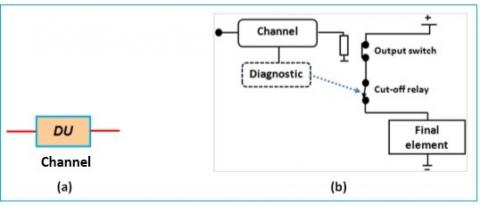Figure 3. (a) Reliability block diagram and (b) basic electrical circuit corresponding to the 1oo1 configuration (with automatic shutdown)

The simple PFH formula for this configuration provided in the standard is:

$P F H_{1001}=\lambda_{D U}$        (4)

3.1.2 Markov model

The multi-phase and approximate Markov models for 1oo1 configuration are respectively shown in Figures 4(a) and (b).

For the multi-phase Markovian model, the probabilities at the beginning of each test period are calculated as follows:

\begin{aligned} \left[\begin{array}{l} P_{1}\left(b_{i+1}\right) \\ P_{2}\left(b_{i+1}\right) \\ P_{3}\left(b_{i+1}\right) \end{array}\right]=&\left[\begin{array}{lll} 1 & 0 & 0 \\ 0 & 1 & 1 \\ 0 & 0 & 0 \end{array}\right]\left[\begin{array}{l} P_{1}\left(e_{i}\right) \\ P_{2}\left(e_{i}\right) \\ P_{3}\left(e_{i}\right) \end{array}\right] \\ & \Rightarrow\left\{\begin{array}{c} P_{1}\left(b_{i+1}\right)=P_{1}\left(e_{i}\right) \\ P_{2}\left(b_{i+1}\right)=P_{2}\left(e_{i}\right)+P_{3}\left(e_{i}\right) \\ P_{3}\left(b_{i+1}\right)=0 \end{array}\right. \end{aligned}              (5)

## 4.png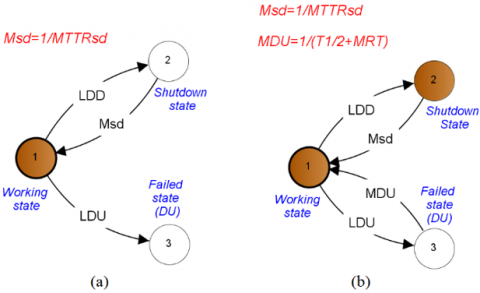Figure 4. Markov models of 1oo1 configuration (a) multi-phase model and (b) classical or approximate model

We notice that the different Markov models provided in this paper are drawn using a dedicated reliability software called GRIF-Workshop . As Greek letters are not allowed within this software, the letters L, M, and B in the Figures stand respectively for λ, μ and β.

3.1.3 PFH formulation

The exploitation of the approximate Markov model allows us to establish the corresponding PFH formula based on the following relation :

$P F H=\sum_{i \in W S} P_{i}(\infty) \sum_{j \in F S} \lambda_{i \rightarrow j}$          (6)

where: WS is the “working state”; FS is the “failed state” and $\lambda_{i \rightarrow j}$  is a failure rate starting from WS and ending in FS. Applying Eq. (6) to 1oo1 configuration gives:

$P F H_{1oo1}=P_{1}(\infty) \lambda_{D U}$          (7)

By determining $P_{1}(\infty)$  from the approximate model, Eq. (7) can be rewritten under the subsequent form:

$P F H_{1oo1}=\left[\frac{\mu_{D U} \cdot \mu_{s d}}{\mu_{D U} \cdot \mu_{s d}+\mu_{D U} \cdot \lambda_{D D}+\mu_{s d} \cdot \lambda_{D U}}\right] \cdot \lambda_{D U}$         (8)

where: $\mu_{D U}=1 /\left(\frac{T_{1}}{2}+M R T\right) \text { and } \mu_{s d}=1 / M T T R_{s d}$ .

As for SIS we can neglect the failures rates vis-à-vis the repair rates ( $\lambda \ll \mu$ ), Eq. (8) can be reduced as follows:

$P F H_{1 o o 1} \approx\left[\frac{\mu_{D U} \cdot \mu_{s d}}{\mu_{D U} \cdot \mu_{s d}}\right] \cdot \lambda_{D U}=\lambda_{D U}$         (9)

One can easily remark that the quantity given by Eq. (9) is the same that provided in Eq. (4). Hence, in the case of 1oo1 architecture, we can validate the IEC 61508 PFH formula even if it is somewhat conservative compared to the accurate one given by Eq. (8).

3.2 2oo2 architecture

3.2.1 Description

It is composed of two identical channels, which means the functioning of both channels is needed for the subsystem to function. The reliability block diagram and the basic electrical diagram corresponding to this configuration are respectively given in Figure 5 (a) and (b). The electrical diagram clearly shows that any DD failure cuts power to the circuit by opening the two diagnostic relays. Therefore, a dangerous state (blocked circuit under voltage) only occurs if at least one of the two channels experiences a DU failure.

## 5.jpg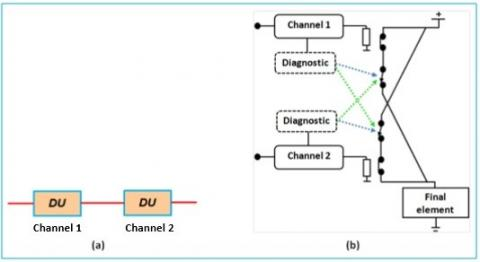Figure 5. (a) Reliability block diagram and (b) basic electrical circuit corresponding to the 2oo2 configuration (with automatic shutdown)

The related IEC 61508 PFH formula is:

$P F H_{2 o o 2}=2 \lambda_{D U}$        (10)

3.2.2 Markov model

Only the approximate Markov model is depicted in Figure 6.

## 6.png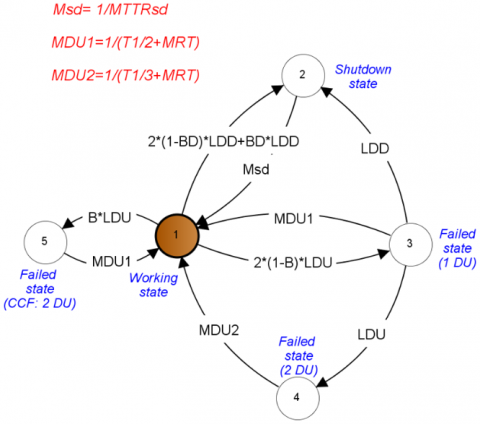Figure 6. Approximate Markov model related to 2oo2 configuration

3.2.3 PFH formulation

The joint use of the above Markov model and Eq. (4) yields:

\begin{aligned} P F H_{2 o o 2}=P_{1}(\infty) & \cdot\left[2(1-\beta) \lambda_{D U}+\beta \lambda_{D U}\right] \\ &=P_{1}(\infty) \cdot(2-\beta) \lambda_{D U} \\ & \approx(2-\beta) \lambda_{D U} \end{aligned}           (11)

The derived PFH formula (Eq. (11)) is slightly different from that of Eq. (10). Regarding the possible values that could be attributed to the factor b, we can validate the IEC formula for this second configuration that maintains the conservative aspect stated for the 1oo1 configuration.

3.3 1oo2 architecture

3.3.1 Description

This configuration is constituted of two identical channels functioning in parallel. It means that the occurrence of a dangerous failure in both channels lead to the failure of the system. According to the shutdown capability, the SIS puts the EUC into a safe state on any detection of a failure in both channels. The reliability block diagram as well as the basic electrical diagram corresponding to this configuration are respectively given in Figures 7 and 8. The electrical diagram shows that cutting off the power to the circuit, in the event of an architecture failure, requires opening the two diagnostic relays. This is only possible with the presence of a DD failure in each channel.

## 7.jpg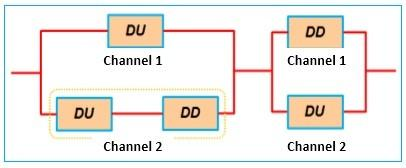Figure 7. Reliability block diagram corresponding to the 1oo2 configuration

## 8.png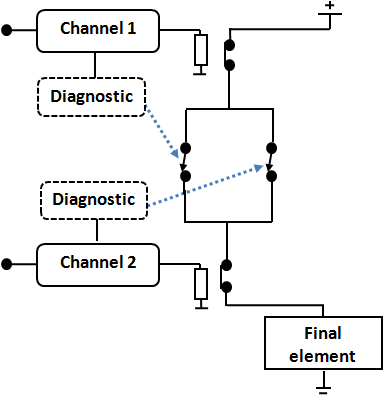Figure 8. Basic electrical circuit corresponding to the 1oo2 configuration

The corresponding PFH formula given in the IEC 61508 standard is reported hereafter:

$\begin{gathered} P F H_{1002}=2 \cdot\left[\left(1-\beta_{D}\right) \lambda_{D D}+(1-\beta) \lambda_{D U}\right] \cdot t_{C E} \\ \cdot(1-\beta) \lambda_{D U}+\beta \lambda_{D U} \end{gathered}$        (12)

where:

$t_{C E}=\frac{\lambda_{D U}}{\lambda_{D}}\left[\frac{T_{1}}{2}+M R T\right]+\frac{\lambda_{D D}}{\lambda_{D}} M T T R$          (13)

3.3.2 Markov model

The corresponding approximate Markov model is given in Figure 9.

## 9.pngFigure 9. Approximate Markov model related to 1oo2 configuration

3.3.3 PFH formulation

Applying Eq. (6) to the above Markov model results in the following PFH formula:

$\begin{gathered} P F H_{1 o o 2}=P_{1}(\infty) \cdot \beta \lambda_{D U}+P_{2}(\infty) \cdot \lambda_{D U}+P_{3}(\infty) \\ \cdot\left[\lambda_{D D}+\lambda_{D U}\right] \end{gathered}$         (14)

The steady state probabilities of occupying the states 1, 2 and 3 are given hereafter.

\left\{\begin{aligned} P_{1}(\infty) & \approx 1 \\ P_{2}(\infty) & \approx \frac{2\left(1-\beta_{D}\right) \cdot \lambda_{D D}}{\mu_{D D}} \\ =2\left(1-\beta_{D}\right) \cdot \lambda_{D D} \cdot M T T R & \\ P_{3}(\infty) & \approx \frac{2(1-\beta) \cdot \lambda_{D U}}{\mu_{D U 1}} \\ =2(1-\beta) \cdot \lambda_{D U}\left(\frac{T_{1}}{2}+M R T\right) & \end{aligned}\right.           (15)

By inserting these quantities in Eq. (14), we obtain the following relation:

\begin{aligned} P F H_{1002} \approx \beta \lambda_{D U} &+2\left(1-\beta_{D}\right) \cdot \lambda_{D D} \cdot M T T R \cdot \lambda_{D U} \\ &+2(1-\beta) \cdot \lambda_{D U} \cdot\left[\frac{T_{1}}{2}+M R T\right] \\ & \cdot\left[\lambda_{D D}+\lambda_{D U}\right] \end{aligned}         (16)

In order to effectively compare formulas given by Eqns. (12) and (16), we rewrite Eq. (16) under a similar form of the formula provided in the IEC 61508 (Eq. (12)). We get:

\begin{aligned} P F H_{oo2} \approx 2 &\left[(1-\beta) \cdot \lambda_{D U} \cdot\left[\frac{T_{1}}{2}+M R T\right]\right.\\ &\left.+\left(1-\beta_{D}\right) \cdot \lambda_{D D} \cdot M T T R\right] \cdot \lambda_{D U} \\ &+\beta \lambda_{D U}+2(1-\beta) \cdot \lambda_{D U} \\ & \cdot\left[\frac{T_{1}}{2}+M R T\right] \cdot \lambda_{D D} \\ =& 2\left[\left(1-\beta_{D}\right) \lambda_{D D}+(1-\beta) \lambda_{D U}\right] \\ & \cdot t_{C E 1} \cdot \lambda_{D U}+\beta \lambda_{D U}+2(1-\beta) \\ & \cdot \lambda_{D U} \cdot\left[\frac{T_{1}}{2}+M R T\right] \cdot \lambda_{D D} \end{aligned}           (17)

where:

$\begin{gathered} t_{C E 1}=\frac{\lambda_{D U}^{i n d}}{\lambda_{D}^{i n d}}\left[\frac{T_{1}}{2}+M R T\right]+\frac{\lambda_{D D}^{i n d}}{\lambda_{D U}^{i n d}} M T T R \\ \lambda_{D D}^{i n d}=\left(1-\beta_{D}\right) \lambda_{D D} ; \lambda_{D U}^{i n d}=(1-\beta) \lambda_{D U} ; \lambda_{D}^{i n d}= \\ \lambda_{D D}^{i n d}+\lambda_{D U}^{i n d} \end{gathered}$          (18)

The examination of Eqns. (17) and (18) shows that the first terms of the summation in Eq. (17) are almost similar to the IEC 61508 PFH formula (Eq. (12)). The tCE given by Eq. (13), as clearly stated in the IEC 61508, is calculated on the basis of 1oo1 configuration, where no CCF is possible. That is why there is no mention of the b factors (b and bD) in Eq. (13). However, the correct quantity is tCE1 given by Eq. (18) because it takes the specificity of the 1oo2 configuration related to the possible occurrence of CCFs. If we disregard the b factors, the first terms of the summation in Eq. (17) would be equal to the PFH formula provided in the IEC 61508. Nevertheless, Eq. (17) contains an additional term: $2(1-\beta) \cdot \lambda_{D U} \cdot\left[T_{1} / 2+M R T\right] \cdot \lambda_{D D}$ . It represents a failure sequence starting with a DU failure and followed by a DD failure: $\text { state } 1 \rightarrow \text { state } 3 \rightarrow \text { state } 7$ (see Figure 9). No possible shutdown due to this sequence, since there is only one DD failure. Therefore, the PFH formula of the standard is formally wrong because it does not consider the abovementioned failure sequence. Hence, the IEC formula would provide underestimated results.

3.4 2oo3 architecture

3.4.1 Description

This configuration is made up of three channels connected in parallel. The functioning of two channels of three is required to ensure the functioning of the system. The reliability block diagram corresponding to this configuration is given in Figure 10, while the associated electrical diagram is presented in Figure 11. The output switches and diagnostic relays are closed during normal operation. The output switches must open in the event of a hazardous situation. Any DD or DU failure would keep these switches closed. With the automatic emergency shutdown capability, DD failures (at least two DD failures) would immediately put the EUC into a safe state, as the corresponding diagnostic relays would open.

## 10.jpg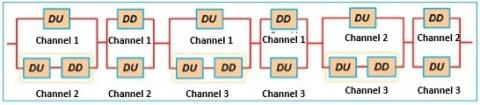Figure 10. Reliability block diagram relating to the 2oo3 configuration

## 11.png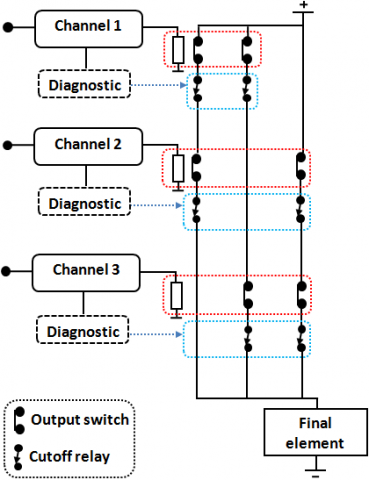Figure 11. Basic electrical diagram relating to the 2oo3 configuration

The corresponding PFH formula given in the IEC 61508 standard is given below:

$\begin{gathered} P F H_{2oo3}=6\left[\left(1-\beta_{D}\right) \lambda_{D D}+(1-\beta) \lambda_{D U}\right] \cdot t_{C E} \\ \cdot(1-\beta) \lambda_{D U}+\beta \lambda_{D U} \end{gathered}$          (19)

3.4.2 Markov model

The corresponding approximate Markov model is given in Figure 12.

3.4.3 PFH formulation

The use of Eq. (6) allows deriving the 2oo3 PFH formula.

\begin{aligned} \mathrm{PFH}_{2oo3} &=P_{1}(\infty) \cdot \beta \lambda_{D U}+P_{2}(\infty) \\ & \cdot\left[2(1-\beta) \lambda_{D U}+\beta \lambda_{D U}\right]+P_{3}(\infty) \\ & \cdot\left[2(1-\beta) \lambda_{D U}+2\left(1-\beta_{D}\right) \lambda_{D D}\right.\\ &\left.+\beta \lambda_{D U}\right] \end{aligned}        (20)

## 12.png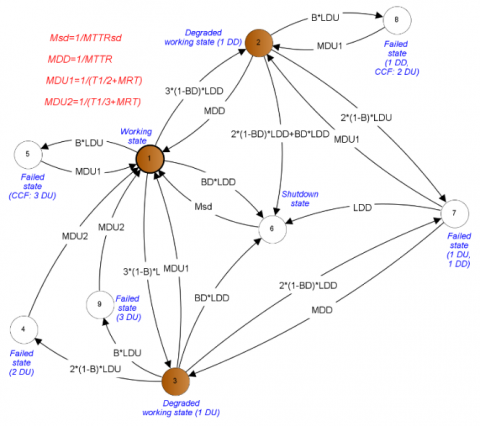Figure 12. Approximate Markov model related to 2oo3 configuration

The steady state probabilities of occupying the states 1, 2 and 3 are given below:

$\left\{\begin{array}{c} P_{1}(\infty) \approx 1 \\ P_{2}(\infty) \approx \frac{3\left(1-\beta_{D}\right) \cdot \lambda_{D D}}{\mu_{D D}} \\ =3\left(1-\beta_{D}\right) \cdot \lambda_{D D} \cdot M T T R \\ P_{3}(\infty) \approx \frac{3(1-\beta) \cdot \lambda_{D U}}{\mu_{D U 1}} \\ =3(1-\beta) \cdot \lambda_{D U}\left(\frac{T_{1}}{2}+M R T\right) \end{array}\right.$          (21)

By inserting the different steady state probabilities and rewriting Eq. (20) under a similar form of Eq. (19), we obtain:

\begin{aligned} P F H_{2 o o 3} \approx 6[&\left.\left(1-\beta_{D}\right) \lambda_{D D}+(1-\beta) \lambda_{D U}\right] \cdot t_{C E 1} \\ & \cdot(1-\beta) \lambda_{D U}+\beta \lambda_{D U}+6(1-\beta) \\ & \cdot \lambda_{D U} \cdot\left[\frac{T_{1}}{2}+M R T\right] \cdot\left(1-\beta_{D}\right) \lambda_{D D} \\ &+3\left(\left(1-\beta_{D}\right) \lambda_{D D} \cdot M T T R\right.\\ &\left.+(1-\beta) \lambda_{D U} \cdot\left[\frac{T_{1}}{2}+M R T\right]\right) \\ & \cdot \beta \lambda_{D U} \end{aligned}          (22)

The same remark made for 1oo2 configuration is still valid regarding the similarity of the first two terms of the summation in Eq. (22) and Eq. (19). In addition, Eq. (22) contains additional terms. It is worth noting that the last summation term of Eq. (22) could be neglected against the second one ( $\beta \lambda_{D U}$ ). However, the third term of the summation, i.e., $6(1-\beta) \cdot \lambda_{D U} \cdot\left[\frac{T_{1}}{2}+M R T\right] \cdot\left(1-\beta_{D}\right) \lambda_{D D}$  cannot be overlooked. Similarly to the case of 1oo2 configuration, this quantity represents a failure sequence starting with a DU failure and followed by a DD failure: $\text { state } 1 \rightarrow \text { state } 3 \rightarrow \text { state } 7$ (see Figure 12). Once again, the PFH formula given in IEC 61508 is formally wrong and would provide underestimated results. Consistency Checking of the IEC 61508 PFH formula for 2oo3 configuration is given in details in the reference .

3.5 1oo3 architecture

3.5.1 Description

This configuration is constituted of three channels connected in parallel. Therefore, the safety function cannot be ensured if a dangerous failure occurs in the three channels. The reliability block diagram corresponding to this configuration is given in Figure 13. The electrical diagram relating to the principle of the 1oo3 architecture, shown in Figure 14, clearly indicates the automatic opening of the electrical circuit following the presence of a DD failure in each of the three channels.

## 13.jpg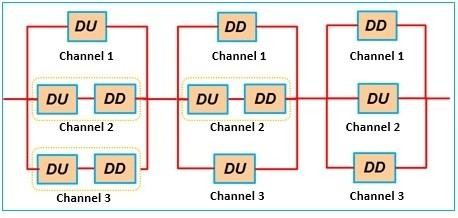Figure 13. Reliability block diagram relating to the 1oo3 architecture

## 14.png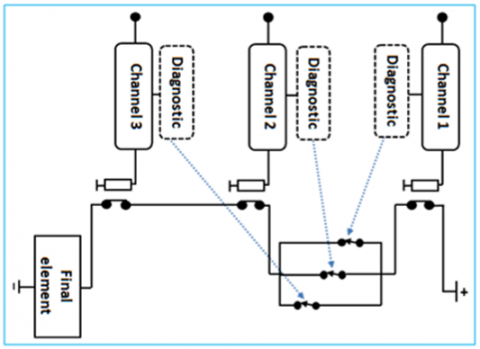Figure 14. Basic electrical diagram relating to the 1oo3 architecture

The IEC 61508 PFH formula for this configuration is:

$\begin{gathered} P F H_{1 o o 3}=6\left[\left(1-\beta_{D}\right) \lambda_{D D}+(1-\beta) \lambda_{D U}\right]^{2} \cdot t_{C E} \\ \cdot t_{G E} \cdot(1-\beta) \lambda_{D U}+\beta \lambda_{D U} \end{gathered}$            (23)

where:

$t_{G E}=\frac{\lambda_{D U}}{\lambda_{D}}\left[\frac{T_{1}}{3}+M R T\right]+\frac{\lambda_{D D}}{\lambda_{D}} M T T R$        (24)

3.5.2 Markov model

The behavior of this latter configuration is given by the approximate Markov model of Figure 15.

\begin{aligned} P F H_{1oo3}=& P_{1}(\infty) \cdot \beta \lambda_{D U}+P_{2}(\infty) \cdot \beta \lambda_{D U} \\ &+P_{3}(\infty) \cdot\left[\beta \lambda_{D U}+\beta_{D} \lambda_{D D}\right] \\ &+P_{4}(\infty) \cdot \lambda_{D U}+P_{5}(\infty) \\ & \cdot\left[\lambda_{D U}+\lambda_{D D}\right]+P_{6}(\infty) \cdot\left[\lambda_{D U}\right.\\ &\left.+\lambda_{D D}\right] \end{aligned}           (25)

3.5.3 PFH formulation

The use of Eq. (6) allows deriving the PFH formula related to the 1oo3 configuration.

The different steady state probabilities are summarized in the following.

$\left\{\begin{array}{l} P_{1}(\infty) \approx 1 \\ P_{2}(\infty) \approx \frac{3\left(1-\beta_{D}\right) \cdot \lambda_{D D}}{\mu_{D D}}=2\left(1-\beta_{D}\right) \cdot \lambda_{D D} \cdot M T T R \\ P_{3}(\infty) \approx \frac{3(1-\beta) \cdot \lambda_{D U}}{\mu_{D U 1}}=2(1-\beta) \cdot \lambda_{D U}\left(\frac{T_{1}}{2}+M R T\right) \\ P_{4}(\infty) \approx \frac{3\left(1-\beta_{D}\right)^{2} \cdot \lambda_{D D}^{2}}{\mu_{D D}^{2}}=3\left(1-\beta_{D}\right)^{2} \cdot \lambda_{D D}^{2} \cdot M T T R^{2} \\ P_{5}(\infty) \approx \frac{6(1-\beta)^{2} \cdot \lambda_{D U}^{2}}{\mu_{D U 1} \cdot \mu_{D U 2}}=6(1-\beta)^{2} \cdot \lambda_{D U}^{2}\left(\frac{T_{1}}{2}+M R T\right)\left(\frac{T_{1}}{3}+M R T\right) \\ P_{6}(\infty) \approx \frac{6(1-\beta) \cdot \lambda_{D U} \cdot\left(1-\beta_{D}\right) \cdot \lambda_{D D}}{\mu_{D U 1} \cdot \mu_{D D}}=6(1-\beta) \cdot \lambda_{D U} \cdot\left(1-\beta_{D}\right) \cdot \lambda_{D D} \cdot\left(\frac{T_{1}}{2}+M R T\right) M T T R \end{array}\right.$            (26)

After inserting the different steady state probabilities and some arrangements, we get:

\begin{aligned} P F H_{1oo3}=6[(1&\left.\left.-\beta_{D}\right) \lambda_{D D}+(1-\beta) \lambda_{D U}\right]^{2} \cdot t_{C E 1} \\ & \cdot t_{G E 1} \cdot \lambda_{D U}+\beta \lambda_{D U} \\ &+6\left[\left(1-\beta_{D}\right) \lambda_{D D}+(1-\beta) \lambda_{D U}\right] \\ & \cdot(1-\beta) \lambda_{D U} \cdot\left[\frac{T_{1}}{2}+M R T\right] \cdot t_{G E 1} \\ & \cdot \lambda_{D D} \\ &+3\left(\left(1-\beta_{D}\right) \lambda_{D D} \cdot M T T R\right.\\ &\left.+(1-\beta) \lambda_{D U} \cdot\left[\frac{T_{1}}{2}+M R T\right]\right) \\ & \cdot \beta \lambda_{D U}+3(1-\beta) \lambda_{D U} \\ & \cdot\left[\frac{T_{1}}{2}+M R T\right] \cdot \beta_{D} \lambda_{D D} \end{aligned}           (27)

where:

$t_{G E 1}=\frac{\lambda_{D U}^{i n d}}{\lambda_{D}^{i n d}}\left[\frac{T_{1}}{3}+M R T\right]+\frac{\lambda_{D D}^{i n d}}{\lambda_{D}^{i n d}} M T T R$            (28)

## 15.png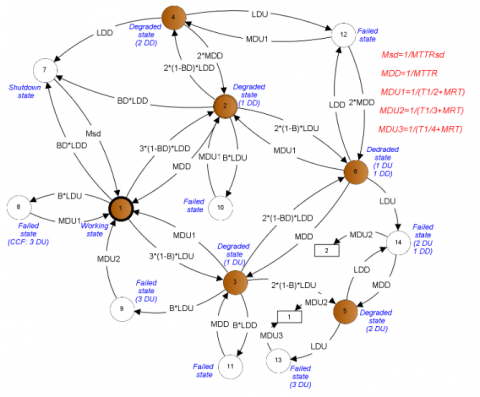Figure 15. Approximate Markov model related to 1oo3 configuration

Similarly to the previous configuration, the examination of Eqns. (23) and (27) shows that their two first summation terms are almost the same. Note that the reasons of the difference between tGE and tGE1 are those stated in relation to tCE and tCE1. Once again, Eq. (27) contains extra terms compared to Eq. (23). Therefore, the PFH formula given in IEC 61508 is formally wrong and would provide optimistic results.

4. Numerical Results

The goal of this section is the numerical verification of the non-validity of the IEC 61508 PFH formulas for some configurations. The verification is obtained using the following approaches: IEC 61508 formulas, Multi-phase Markov models (MPM), approximate Markov models (AM) and the new derived formulas. Note that the numerical results associated with the developed MPM and AM models are obtained using GRIF-Workshop . The used parameters are: λD = 5E-6 h-1; MTTR = MRT = 8 h; T1 = 4380 h; $\beta=2 \beta_{D}=0.1$; MTTRsd = 24 h. Different values for DC are used.

4.1 1oo1 and 2oo2 configurations

The obtained results for these configurations are respectively gathered in Tables 1 and 2.

Table 1. PFH Results for 1oo1 configuration

 DC Approaches IEC: Eq. (4) MPM AM Eq. (9) 0.6 2E-6 1.991E-6 1.992E-6 2E-6 0.9 5E-7 4.994E-7 4.994E-7 5E-7 0.99 5E-8 4.999E-8 4.999E-8 5E-8

Table 2. PFH Results for 2oo2 configuration

 DC Approaches IEC: Eq. (10) MPM AM Eq. (11) 0.6 4E-6 3.768E-6 3.769E-6 3.8E-6 0.9 1E-6 9.478E-7 9.479E-7 9.5E-7 0.99 1E-7 9.496E-8 9.496E-8 9.5E-8

The inspection of Table 1 shows that the PFH results derived from the MPM and AM approaches are almost identical. In addition, they are very close to the results given by analytical formulas (Eqns. (4) and (9)), which are slightly conservative.

Table 2 shows that the PFH results obtained from the MPM, AM and Eq. (11) are very close to each other. The results induced by Eq. (10) (IEC 61508 formula) are higher than the previous ones. For the cases of DC = 0.9 and 0.99, the results related to the IEC formula induce a SIL2, whereas the other approaches lead to a SIL3 (according to the IEC 61508 SIL table). Despite this discrepancy, the IEC formula is conservative and does not underestimate the SIL of the 2oo2 configuration.

For these two first configurations, the IEC 61508 standard provides acceptable formulas which provide conservative results compared to the accurate ones determined from the MPM and AM models.

4.2 1oo2, 2oo3 and 1oo3 configurations

In order to carry out an effective comparison between the different approaches, we only consider the contribution of independent failures: $\beta=2 \beta_{D}=0$. Actually, the common term ($\beta \lambda_{D U}$) between the IEC formulas and new ones related to common cause failures may overwhelm the PFH results.   The obtained results for these configurations are respectively shown in Tables 3, 4 and 5.

Table 3. PFH Results for 1oo2 configuration without CCF

 DC Approaches IEC: Eq. (12) MPM AM Eq. (17) 0.6 1.768E-8 4.357E-8 4.348E-8 4.406E-8 0.9 1.135E-9 1.096E-8 1.099E-8 1.103E-8 0.99 1.495E-11 1.099E-9 1.102E-9 1.103E-9

Table 4. PFH Results for 2oo3 configuration without CCF

 DC Approaches IEC: Eq. (19) MPM AM Eq. (22) 0.6 5.304E-8 1.299E-7 1.293E-7 1.322E-7 0.9 3.405E-9 3.285E-7 3.289E-7 3.308E-7 0.99 4.485E-11 3.295E-9 3.307E-9 3.309E-9

Table 5. PFH results for 1oo3 configuration without CCF

 DC Approaches IEC: Eq. (23) MPM AM Eq. (27) 0.6 1.570E-10 3.818E-10 3.808E-10 3.912E-10 0.9 2.622E-12 2.508E-11 2.523E-11 2.547E-11 0.99 5.068E-15 3.699E-13 3.724E-13 3.739E-13

The examination of Tables 3, 4 and 5 shows that the results determined using the MPM, AM and new formulas are very close. The results obtained from the new formulas are very slightly conservative. The IEC formulas, which are formally wrong as demonstrated in section 3, induce lower results compared with those obtained from the other approaches. Therefore, the IEC formulas could lead to underestimated SIL, which is dangerous from a safety point of view. It should be noted that the obtained results do not consider the contribution of CCFs that would reduce the discrepancies between the results of the IEC formulas and the ones related to the other approaches. However, even with the consideration of CCFs, the IEC formulas could results in wrong SILs.

5. Conclusions

Safety instrumented systems constitute a vital safety barrier for controlling the occurrence of hazardous events. The main objective of this paper was to check the validity of the IEC 61508 standard related to the PFH measure with shutdown capability. For this end, the safety system configurations addressed in this standard have been modeled using Markov models (multi-phases and approximate models). New PFH formulas have been derived from the approximate models. The examination of these formulas showed that the IEC formulas are only valid for the case where the number of DD failure leading to a shutdown state N-K+1=1 (1oo1 and 2oo2 configurations). This remark could be generalized to the NooN system. For $\mathrm{N}-\mathrm{K}+1 \neq 1$, the new formulas contain extra terms compared to the IEC formulas. Thus, these latter formulas induce an underestimated PFH results which is dangerous from a safety point of view. This fact was confirmed through different numerical comparisons.

This paper does not consider generalized formulas (for any KooN configuration) for the PFH measure with shutdown capability. This limitation will be addressed in a future work.

Nomenclature
 AM Approximate Markov model CCF Common causes Failure DC Diagnostic coverage for dangerous failure DD Dangerous detected DU Dangerous undetected EUC Equipment under control FE Final element LS Logic solver MPM Multi-phase Markov model MRT Mean repair time (for DU failures) MTTR Mean time to restoration (for DD failures) MTTRSD Mean duration to restart after shutdown PFDavg Average probability of dangerous failure on demand PFH Probability of dangerous failure per hour S Sensing element SIF Safety instrumented function SIL Safety integrity level SIS Safety instrumented systems Symbols $\beta$ CCF proportion for DU failures $\beta_{D}$ CCF proportion for DD failures λD Dangerous failure rate $\lambda_{D D}$ DD failure rate $\lambda_{D D}^{i n d}$ Independent DD failure rate $\lambda_{D D}^{C C F}$ Dependent DD failure rate $\lambda_{D U}$ DU failure rate $\lambda_{D U}^{i n d}$ Independent DU failure rate $\lambda_{D U}^{C C F}$ Dependent DU failure rate T1 Proof tests interval
References

 IEC61508. (2010). Functional safety of electrical/electronic/programmable electronic safety-related systems. Parts1 to 7. 2nd ed. Geneva: International Electrotechnical Commission.

 IEC 61511. (2016). Functional Safety-Safety Instrumented Systems for the Process Industry Sector Parts 1 to 3. 2nd ed. Geneva: International Electrotechnical Commission.

 ANSI/ISA 84.00.01-2004. (2004). Functional safety: Safety instrumented systems for the process industry sector. New York City: International Society of Automation, Research Triangle Park.

 CCPS. (2016). Guidelines for safe automation of chemical processes. 2nd ed. New Jersey: AIChE/CCPS John Wiley & Sons, Inc., Hoboken.

 CCPS. (2017). Guidelines for safe and reliable instrumented protective systems. New Jersey: AIChE/CCPS John Wiley & Sons, Inc., Hoboken.

 Hauge, S., Lundteigen, M.A., Hokstad, P., Håbrekke, S. (2010). Reliability Prediction Method for Safety Instrumented Systems-PDS Method Handbook, 2010 edition. SINTEF report STF50 A, 6031, 460.

 Innal, F. (2008). Contribution to modelling safety instrumented systems and to assessing their performance. Critical analysis of IEC 61508 Standard, Ph. D. thesis, Engineering.

 Chebila, M., Innal, F. (2015). Generalized analytical expressions for safety instrumented systems’ performance measures: PFDavg and PFH. Journal of Loss Prevention in the Process Industries, 34: 167-176. http://dx.doi.org/10.1016/j.jlp.2015.02.002

 Hokstad, P., Rausand, M., (2008). Hanbook of performability engineering: Common cause failure modeling: Status and trends. In: K.B. Misra, London: Springer, pp. 621-640. https://doi.org/10.1007/978-1-84800-131-2_39

 Chebila, M., Innal, F. (2014). Unification of common cause failures’ parametric models using a generic markovian model. Journal of Failure Analysis & Prevention, 14(3): 426-434. https://doi.org/10.1007/s11668-014-9828-0

 Omeiri, H., Hamaidi, B., Innal, F., Liu, Y. (2020). Verification of the IEC 61508 PFH formula for 2oo3 configuration using Markov chains and Petri nets. International Journal of Quality & Reliability Management, 38(2): 581-601. https://doi.org/10.1108/IJQRM-09-2019-0305

 Dutuit, Y., Rauzy, A., Signoret, J.P. (2008). A Snapshot of methods and tools to assess safety integrity levels of high-integrity protection systems. Proceedings of the Institution of Mechanical Engineers, Journal of Risk & Reliability, 222(3): 371-379. https://doi.org/10.1243/1748006XJRR147

 Langeron, Y., Barros, A., Grall, A., Bérenguer, C. (2008). Combination of safety integrity levels (SILs): A study of IEC 61508 merging rules. Journal of Loss Prevention in the Process Industries, 21(4): 437-449. https://doi.org/10.1016/j.jlp.2008.02.003

 Mechri, W., Simon, C., Bicking, F., BenOthman, K. (2013). Fuzzy multiphase Markov chains to handle uncertainties in safety systems performance assessment. Journal of Loss Prevention in the Process Industries, 26(4): 594-604. https://doi.org/10.1016/j.jlp.2012.12.002

 Goble, W.M. (1998). The use and development of quantitative reliability and safety analysis in new product design. PhD thesis. Eindhoven University of Technology, Netherland.

 Charpentier, P. (2002). Architecture d'automatisme en sécurité des machines: Etudes des conditions de conception liées aux défaillances du mode commun. PhD thesis. National Polytechnic Institute of LORRAINE, France.

 GRIF-Workshop. (2018). Graphical interface for reliability forecasting software. Available at: http://grif-workshop.com.

 Omeiri, H., Innal, F., Hamaidi, B. (2015). Safety integrity evaluation of a butane tank overpressure evacuation system according to IEC 61508 standard. Journal of Failure Analysis & Prevention, 15(6): 892-905. https://doi.org/10.1007/s11668-015-0031-8